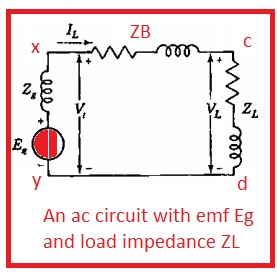Hello, readers welcome to a new post in this post we will discuss the Difference between Single Subscript and Double Subscript Notation. To see the value of voltage among the reference point in any circuitry there is the usage of a single subscript notation. Certain point is taken as before the application of single or double notation to any circuitry to finds different parameters.

In this post, we discuss the different parameters related to single subscripts and double subscripts with the details. So let get started

### What is Single Subscript Notation

• In the below diagram, we can see circuitry that is an alternating current circuit, and the voltage source is linked with it.• The voltage source linked is denoted as Eg and voltage among the two nodes is X and y is denoted with Vt. the current is passing in the circuitry is denoted as IL or load current and voltage value about the ZL is denoted as VL.
• For an indication of polarity, there is a plus and minus sign ia drawn to the circuit.
• In the case of alternating current circuitry, the point denoted with plus sign indicates that voltage is positive according to other points.
• In the above circuitry, the instantaneous voltage Vt has a positive value in the case of the point mentioned with postive and indicates its value of voltage is high the negative point.
• In some cases, there is arrowhead used to show the polarity of current and the direction head of the arrow indicates that in this direct current has a positive value.
• The value of phasor current for the above circuitry is mentioned here.

IL=(Vt+VL)/ZB

Vt=Rg-ILZg

• Each node is denoted with letters and voltage for every node can e denoted with a certain letter according to the reference node.
• The instantaneous voltage vx and phasor voltage Vx explain the voltage for node denoted with X according to reference node Y the value of Vx is positive in case X has high potential than Y.
• In result.
• vx= vt and Vx=Vt

### Wha is Double Subscript Notation

• Contrary to single subscript notation there is no use of polarity marks and arrows head in the double subscript notation.
• The explanation of three-phase circuits is done through use of double subscript notation
• In the above circuitry, the direction of the arrow from X to C denotes the positive current direction for IL. The value of instantaneous current is positive from X to C and for double subscript notation the current value is ixc.
• The value of current ixc is equal to -icx.
• In the case of double subscript, the letters show the voltage for specific nodes.
• In other words voltage about Zl is VCD according to o nodes c and d as circuit is ac so for positive half cycle when C is at high potential then d Vcd is positive.
• The phasor voltage is VCD according to the current ICD moving from node C to D.

Vcd=IcdZA

Icd=YAVcd

• ZA denotes the complex impedance and YA is the complex admittance that is inverse of complex impedance.
• By changing the sequence of subscript forr current and voltage for one-eighty degrees from real phase we have

Vdc=Vcd£^j180=Vcd∠180=-Vcd

• The relation among the single and double subscript notation for the below circuitry is given here.Vt=Vx=Vxy

VL=VC=VCy

IL=ICd

• By using KVl we have

Vyx+Vxc+Vcd

So that is all about the Difference between Single Subscript and Double Subscript Notation if you have any further query ask in the comments. Thanks for reading have a nice day